Function Repository Resource:

# GyrationTensor

Compute the gyration tensor and derived metrics quantifying the shape of particle distributions

Contributed by: Nicholas E. Brunk, Wolfram|Alpha Math Team
 ResourceFunction["GyrationTensor"][{p1,p2,…}] returns the gyration tensor of points {p1,p2,…}. ResourceFunction["GyrationTensor"][{p1,p2,…},type] returns the derived metric specified via type.

## Details

ResourceFunction["GyrationTensor"] allows points {p1,p2,}of any consistent dimensionality n.
Metric type availability depends upon the dimensionality of the coordinates in n:
 Dimension n type any "ResourceFunction["GyrationTensor"]", "RadiusOfGyration", "Eigenvalues" 2 "Acircularity", "NormalizedAcircularity" 3 "Asphericity", "NormalizedAsphericity", "RelativeAnisotropy" >3 "HyperAsphericity", "NormalizedHyperAsphericity"

## Examples

### Basic Examples (1)

By default, GyrationTensor returns the tensor itself:

 In:=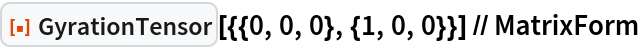Out=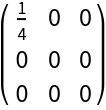### Scope (2)

Other metrics derived from the gyration tensor may be computed:

 In:=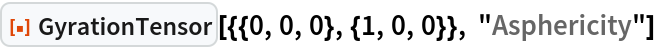Out=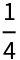Metrics may be computed in other dimensions, for example 2D and 4D, respectively:

 In:=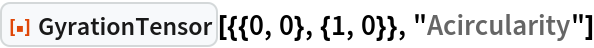Out=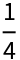In:=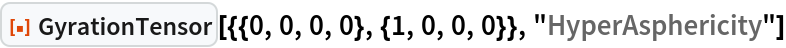Out=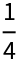### Properties and Relations (2)

The metrics "NormalizedAsphericity" and "RelativeAnisotropy" have the same limiting behavior in describing spherically symmetric vs. linear distributions:

 In:=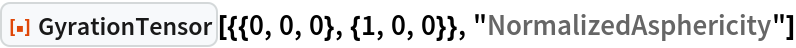Out=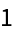In:=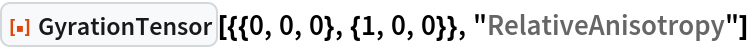Out=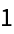These metrics do, however, differ in non-limiting cases:

 In:=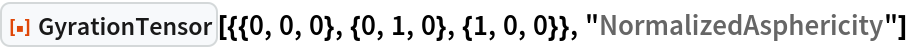Out=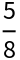In:=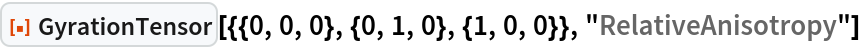Out=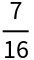### Possible Issues (2)

Available metrics are determined by the dimensions of the input vectors, such that "Acircularity"—a 2D metric—is not computed for 3D:

 In:=Out=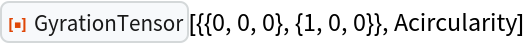A given dimension can be omitted to check symmetry in the remaining fewer dimensions:

 In:=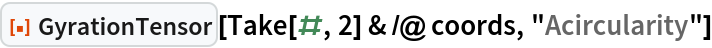Out=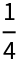Non-normalized metrics scale with the size of the distribution:

 In:=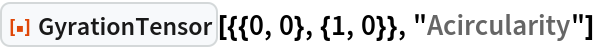Out=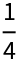In:=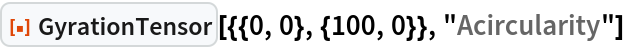Out=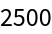Normalized equivalents do not and are scaled between 0 and 1:

 In:=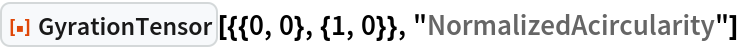Out=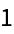In:=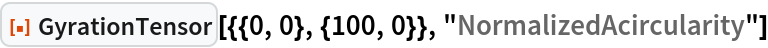Out=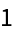### Neat Examples (8)

Closely approximate the radius of gyration of a given 2D region, for example, reproducing known quantities such as a hoop (unit circle):

 In:=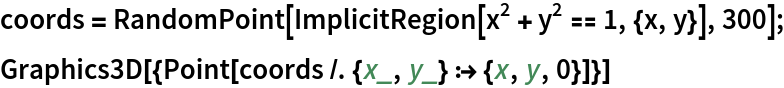Out=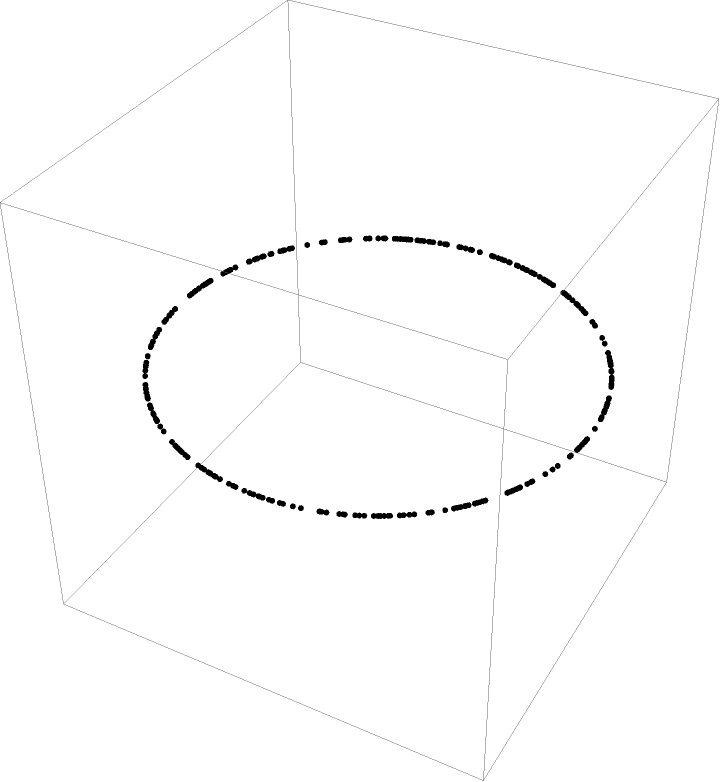In:=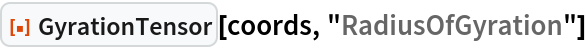Out=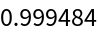The same works for a hollow, 3D unit sphere:

 In:=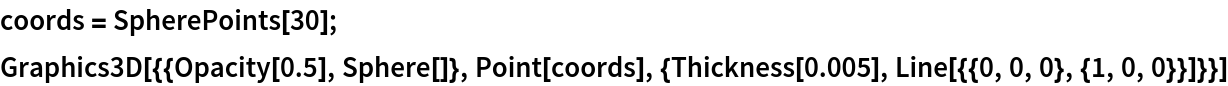Out=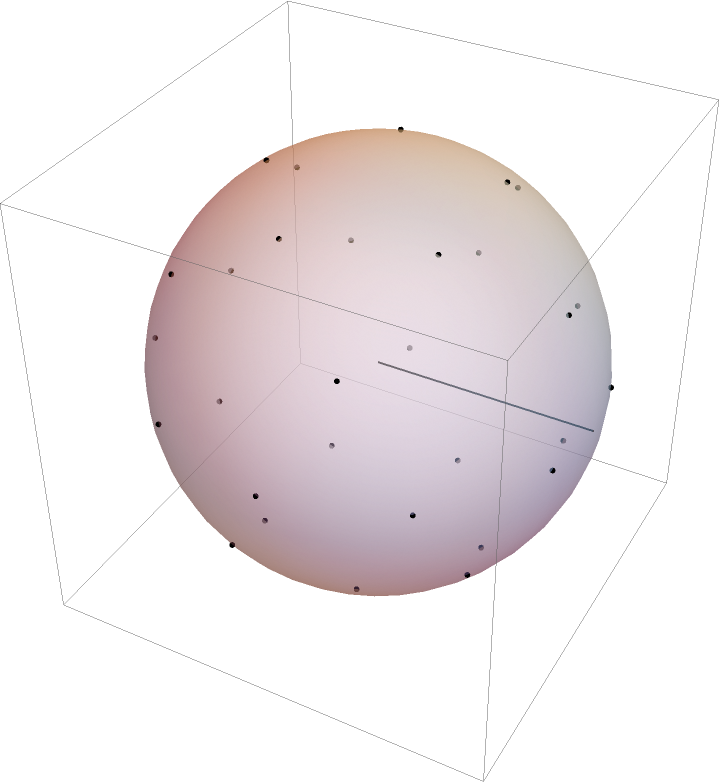The hollow sphere has a known radius of gyration Rg=R=1, which can be approximated (quite accurately, given the SpherePoints function):

 In:=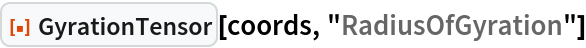Out=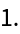The same works for a solid, 3D unit sphere, using ImplicitRegion:

 In:=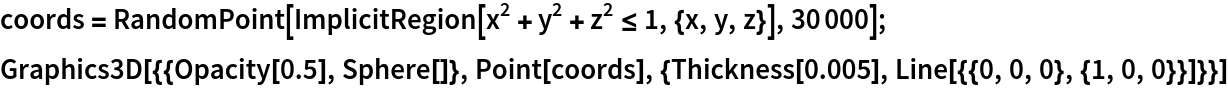Out=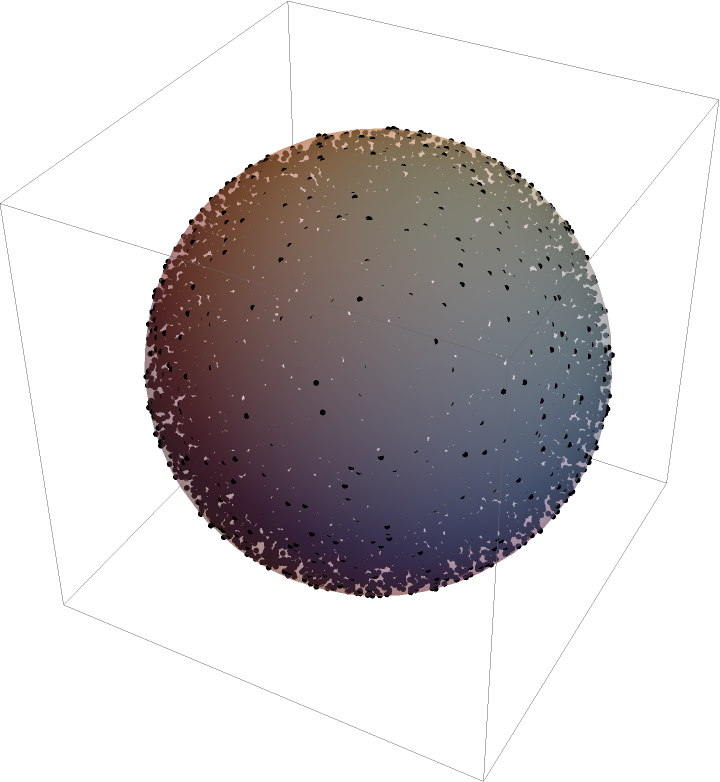The solid sphere has a known radius of gyration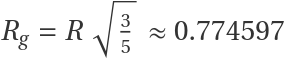:

 In:=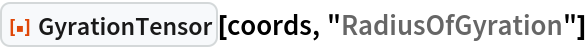Out=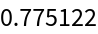The intermediate result of a hollow spherical shell of a given thickness may also be estimated, using ImplicitRegion:

 In:=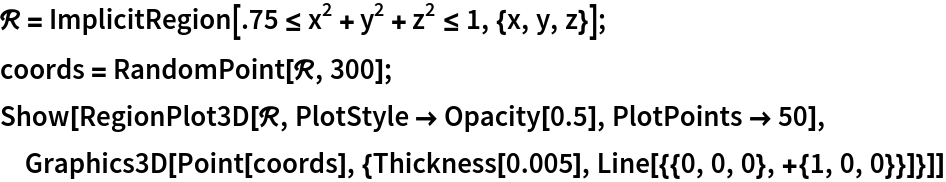Out=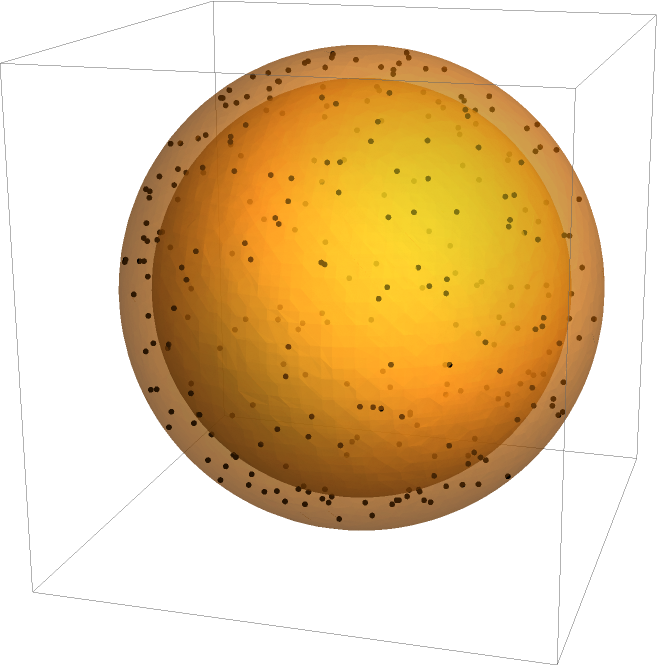The radius of gyration may be estimated:

 In:=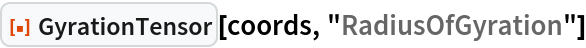Out=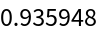This result can be verified, in that it converges on the previous answer for the perfectly hollow unit sphere as thickness t0:

 In:=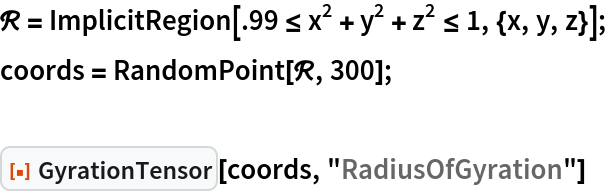Out=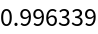The same works for a solid, 3D cylinder, e.g. with unit radius and length, for which a Region may be defined from a pre-existing graphics primitive:

 In:=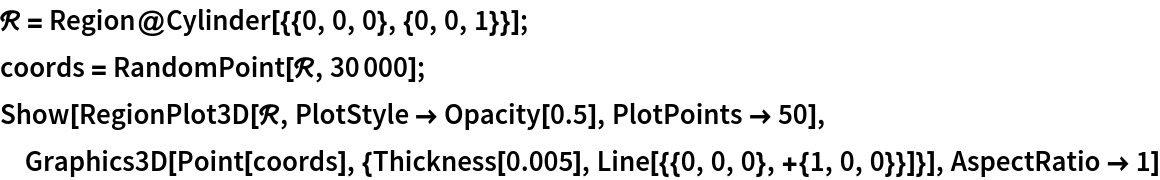Out=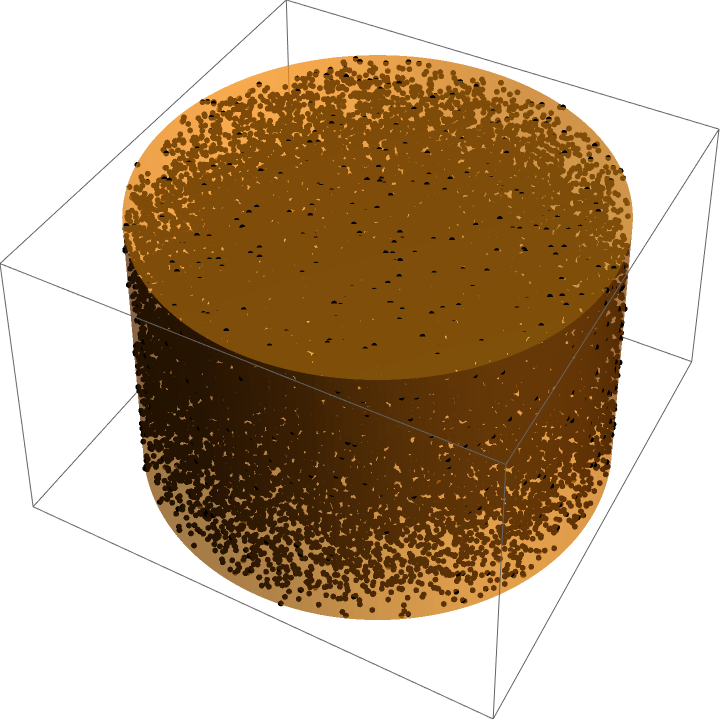A solid cylinder has a known radius of gyration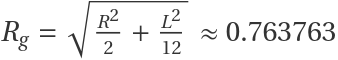:

 In:=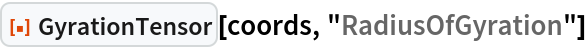Out=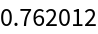Estimate the radius of gyration of a rabbit from ExampleData:

 In:=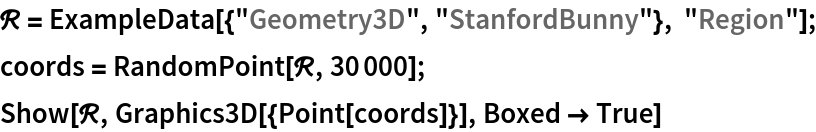Out=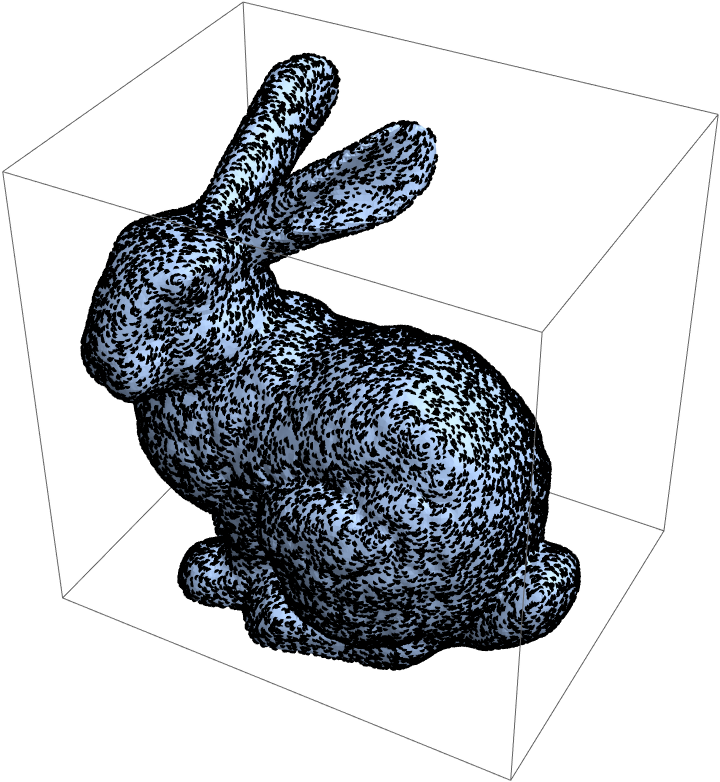Note that this is a hollow rabbit, so it is more relevant to the Easter chocolate shell version than a live rabbit:

 In:=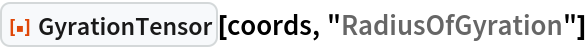Out=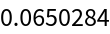Shape metrics derived from the gyration tensor may be used to characterize the shape of a random walk. An unbiased random walk will generally have lower normalized asphericity than a biased random walk:

 In:=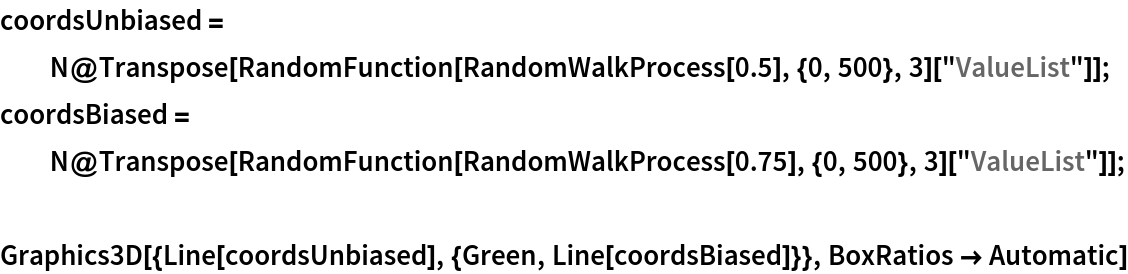Out=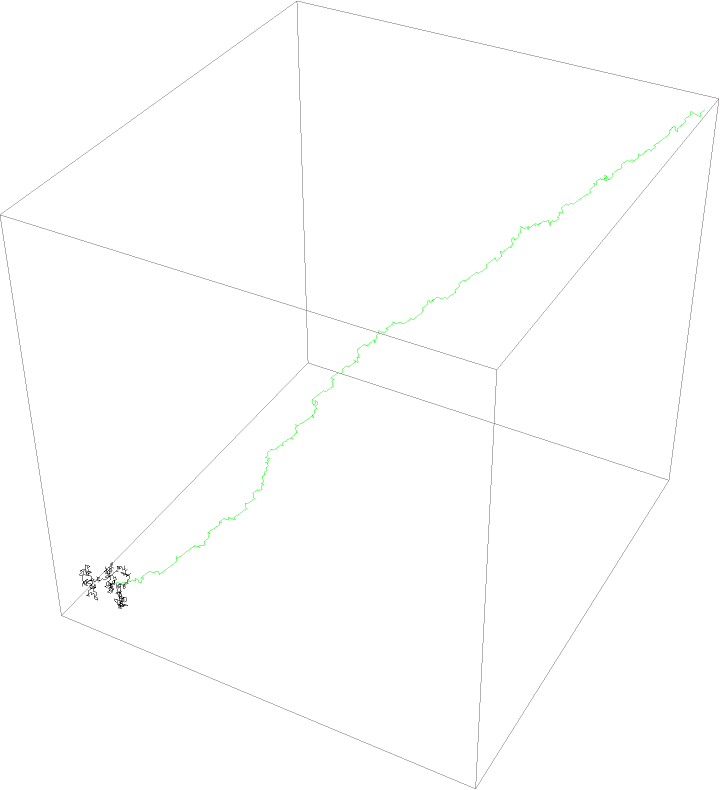The unbiased walk (black) is much more spherically symmetric than the predominantly linear, biased random walk (green):

 In:=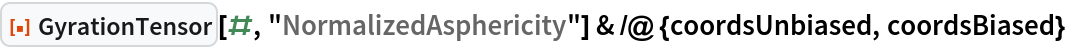Out=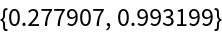The relative anisotropy may be used to show that caffeine is a less linear molecule than THC:

 In:=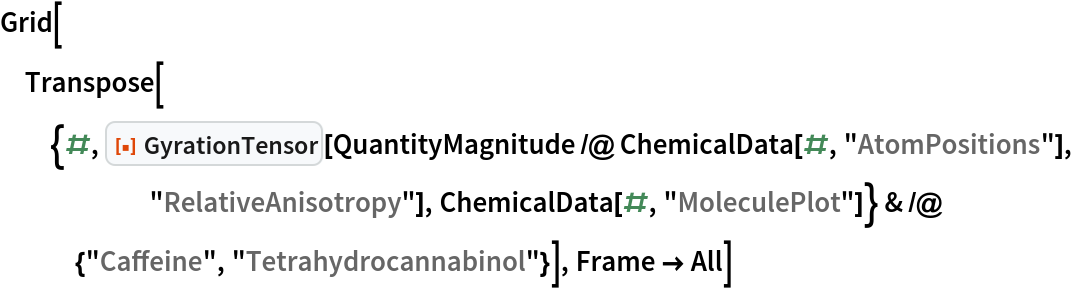Out=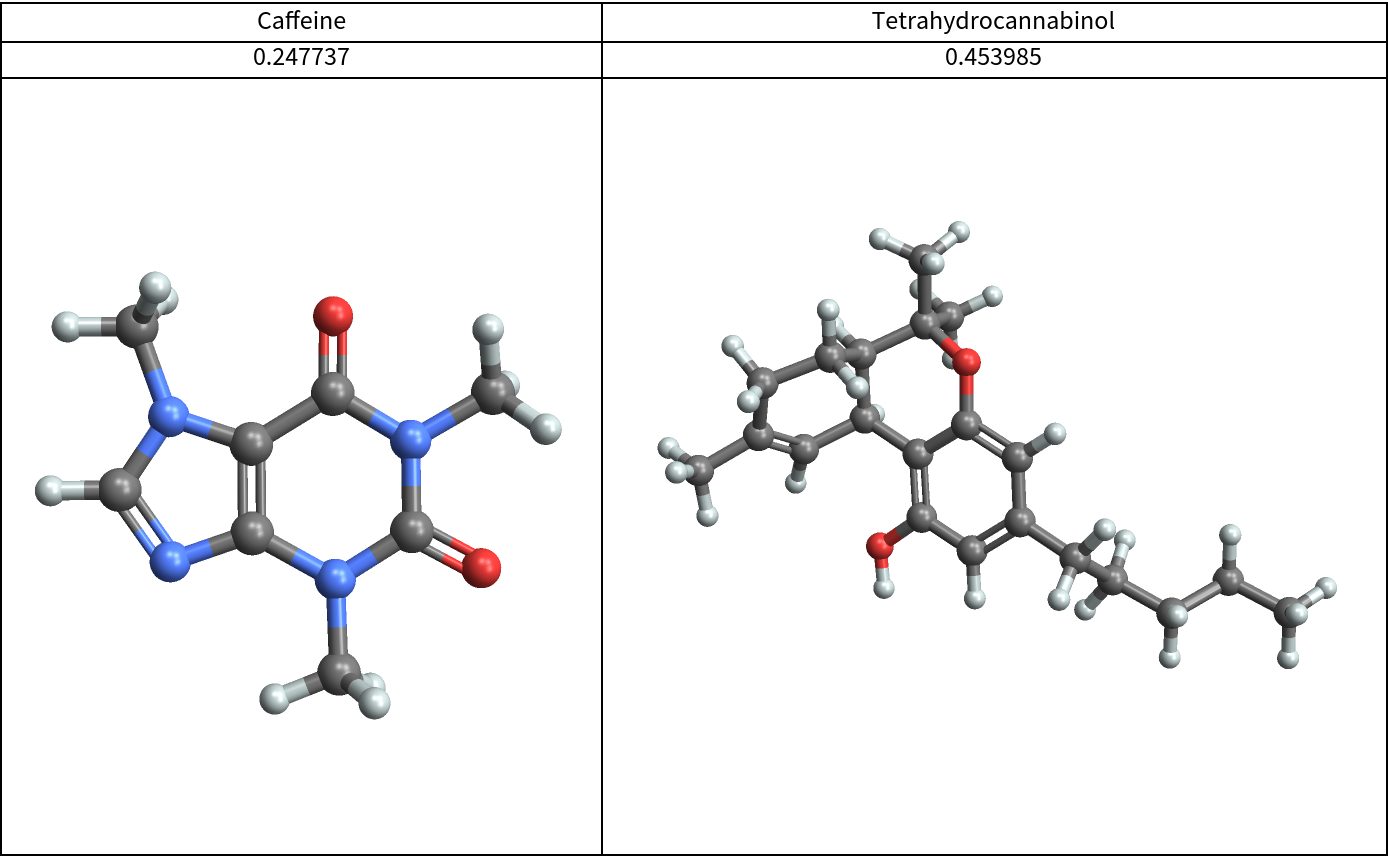## Publisher

Wolfram|Alpha Math Team

## Version History

• 2.0.0 – 23 March 2023
• 1.0.1 – 16 March 2021
• 1.0.0 – 18 September 2020